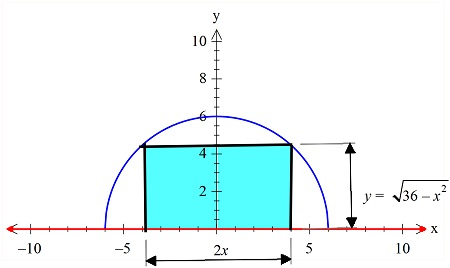# A rectangle is bounded by the x - axis and the semicircle y^2 = 36 - x^2 . Write the...

## Question:

A rectangle is bounded by the {eq}x {/eq} - axis and the semicircle {eq}y^2 = 36 - x^2 {/eq}. Write the area {eq}A(x) {/eq} of the rectangle as a function of {eq}x {/eq}, and determine the domain of the area function.

## Area:

The area of the semi-circle given in the problem and the area of the rectangle that is inscribed within it will be related to each other. If the area function is to be found with any of the axes, then we can just choose the expression for length and width.

We have plotted the graph of the semi-circle and the rectangle whose area is given in blue color.Now this area is given by the expression:

{eq}A(x)=y (2x)=2x \sqrt{36-x^2} \\ {/eq}

Also, it can be seen that the domain of x will be from:

-6 to 6, as beyond this the rectangle will not be withing the semi-circle.